# Six Sigma DMAIC Process - Measure Phase - Measurement System

The Objective of this section is to identify and understand the components of variation arising out of the measurement system and to be able to use the appropriate tool for analysis depending on the data type.

Measurement System Analysis (MSA) for Data Types: For continuous data type the Gage Repeatability and Reproducibility (R&R) studies are done and we check for
• % Tolerance
• % Contribution
• Number of Distinct Categories
For discrete data the Discrete Data Analysis (DDA) is done
• Accuracy
• Repeatability
• Reproducibility
All of the above values are derived out of any statistical software. We will see a few thumb of rules after understanding the Measurement System in more detail.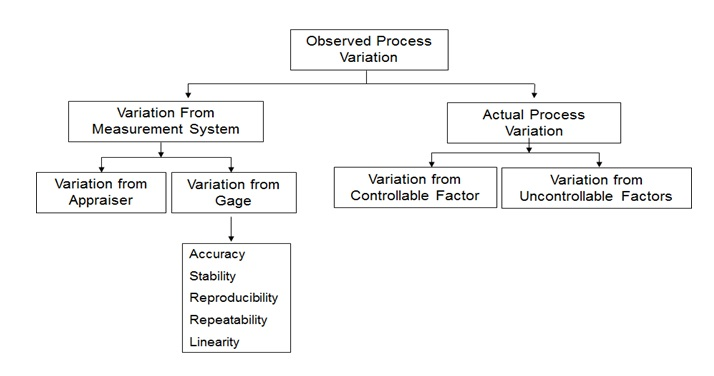Six Sigma Components of Variation

The variation in process can result due to the Actual Process Variation and the Variation from Measurement System. The Actual Process Variation is resulted because of Controllable Factors and/or Uncontrollable Factors.

Variation from Measurement System is not advisable. This variation is due to Variation from Appraiser (due to the operator – individual who operates the tool/instrument/gate) and Variation from Gage i.e. Variation due to the instrument itself. Variation from gage can be further classified into Accuracy, Stability, Repeatability, Reproducibility and Linearity.

Variation (observed) = Variation (Actual) + Variation (Measurement)

The above equation indicates that the Observed Variation in a process is due to Actual Variation and the variation due to the Measurement System.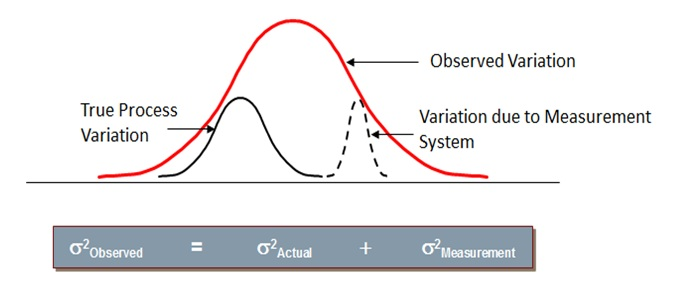Six Sigma Process Variation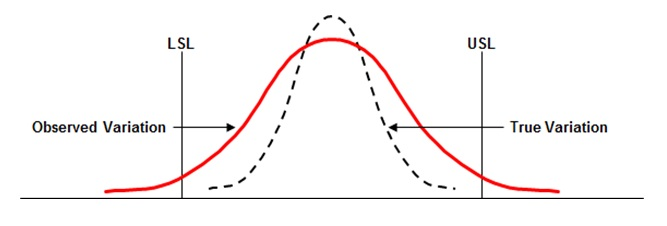Six Sigma Observed Variation vs True Variation

In the above example, LSL – Lower Specification Limit and USL – Upper Specification Limit.

Measurement System Analysis:
Measurement system errors can be due to
• Accuracy – The difference between the average of observed values and the standard
• Repeatability – Variation in measurement when a person measures the same unit repeatedly with the same measuring gage (or tool)
• Reproducibility - Variation in measurement when two or more persons measure the same unit using the same measuring gage (or tool)
• Stability - Variation in measurement when the same person measures the same unit using the same measuring gage (or tool) over an extended period of time.
• Linearity – The consistency of the measurement across the entire range of the measuring gage.
Acceptable Level of Measurement System Variation (Thumb of rules):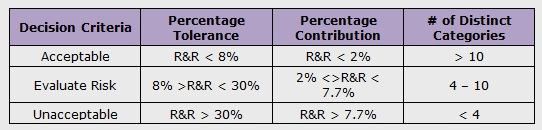Six Sigma Gage R&R Thumb Rule – Continuous DataSix Sigma Attribute R&R Thumb Rule – Discrete Data

Process Variation:
Process Variation is generally due to two causes: Common Causes and Special Causes. Common cause is also called as Noise. Variation is inherent in the system. It results in a stable – IN CONTROL – process because the variation is predictable and it is evident in the system. Whereas Special causes are also called as Signals. They are unexpected occurrences due to unforeseen circumstances. It results in an unstable – OUT OF CONTROL – process because the variation is not predictable.

Data Interpretation:
Data is interpreted using factors such as Stability, Normality, Shape, Spread and Centering.

Let’s take a look at the first factor – Stability. Run chart is an important tool for understanding data stability. Run charts are simple time ordered plots of process data. On run chart plots one can perform tests for certain patterns in the data to understand data stability and presence of these patterns indicate special causes of variation.

Special cause variation in a run chart can be understood by the following patterns: Same Value Plot, Clustering or Too Few Runs Plot, Mixtures or Too Many Runs Plot, Oscillations Plot, Trends Plot, Shifts Plot.

Run Chart – Same Value Plot:
A run chart having seven or more points with same value in a sequence indicates bias in the process.Six Sigma Run Chart – Same Value Plot

Run Chart – Clustering Plot:
Such a plot has lot of data points next to each other on one side of the median and the same way on the side of the median over time and indicates a periodic shift in process average due to lot-to-lot or setup variability; Also known as too few runs plot.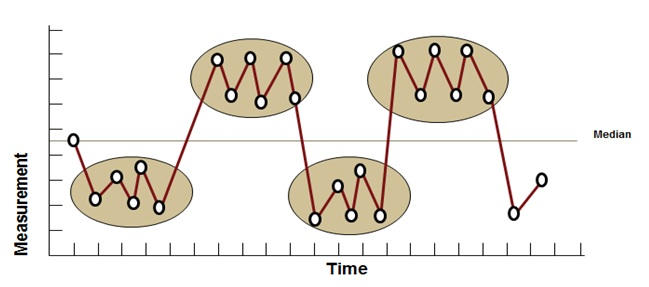Six Sigma Run Chart – Clustering Plot

Run Chart – Mixtures Plot:
Such a plot has and absence of points near the center line with a sequence of fourteen or more points in a row alternating up and down; indicates a bias or systematic sampling from different sources or processes; Also known as cycle or too many runs plot.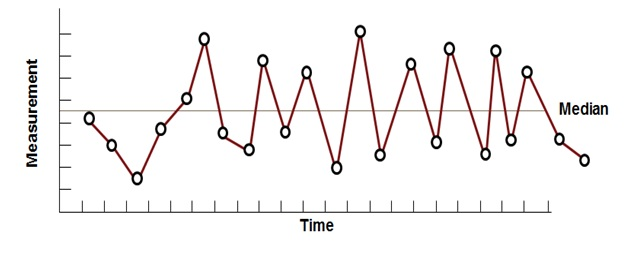Six Sigma Run Chart – Mixtures Plot

Run Chart – Oscillation Plot:
Such a plot, has data points constantly fluctuating up and down rapidly around the median and indicates that the process is not steady or stable.Six Sigma Run Chart – Oscillation Plot

Run Chart – Trend Plot:
Such a plot has a sequence of seven or more data points continuously increasing or decreasing and indicates a gradual increase or decrease trend in the data measurement methods.Six Sigma Run Chart – Trend Plot

Run Chart – Shift Plot:
Such a plot has a sequence of eight or more points on the same side of the median and indicates a gradual shift in the process.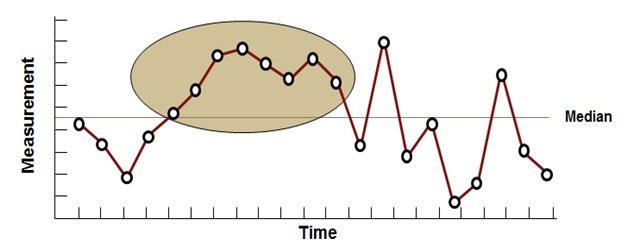Six Sigma Run Chart – Shift Plot

Introduction to Normal Distribution:
Normal Distribution was developed by astronomer Karl Gauss. It is a most prominently used distribution in statistics. Its applicability is to many situations where given the population knowledge, we need to predict the sample behavior. It comes close to fitting the actual frequency distribution of many phenomena such as:
• Human characteristics such as weights, heights & IQ’s
• Physical process outputs such as yields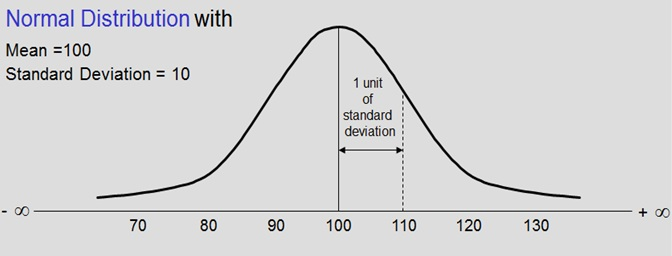Six Sigma Normal Distribution - 1

It’s a Probability Distribution, illustrated as N ( µ, σ ) i.e. it is characterized by Mean and Standard Deviation. Simply put, a probability distribution is a theoretical frequency distribution. It has higher frequency of values around the mean & lesser & lesser at values away from mean. It is Continuous & Symmetrical. Its tails are asymptotic to X-axis i.e. the tails will never touch the X-axis. It is Bell shaped and the Total area under the Normal curve = 1.

For the data that does not follow normal distribution, the data will not be bell shaped as above. It could be stretched to the left or right or it can also have multiple peaks or it may show some other pattern. We term such data as non-normal data.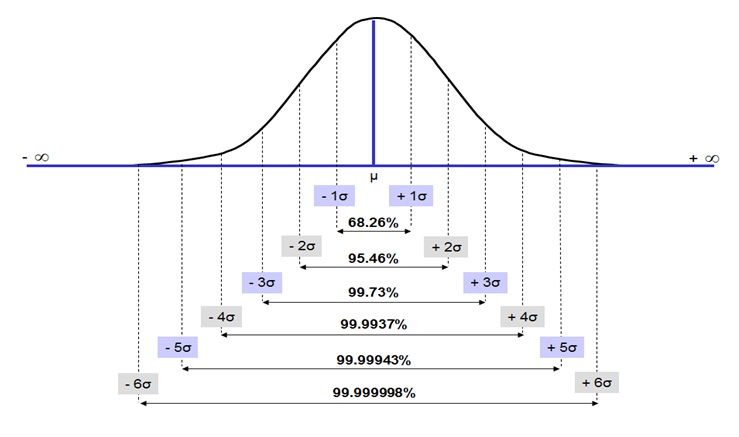Six Sigma Normal Distribution - 2

As you will observe in the above diagram, a process with 1σ (one sigma), the performance of the process is at 68.26%. As the Sigma value increases (2 σ, 3 σ, 4 σ, etc), the performance of the process also increases (95.46%, 99.73%, 99.9937%, etc). When the process achieves 6 σ level, the performance of the process is as high as 99.999998%.

Now let us look at how we can determine Normality of the data set. Anderson-Darling normality test is performed on the data to understand its normality characteristics. A data is considered to be normal if the P-value is greater than .05, and the hypothesis test states:
• H0 – Data is normal
• Ha – Data is not normalSix Sigma Histogram Distribution Symmetrical ShapeSix Sigma Histogram Distribution Positively Skewed Shape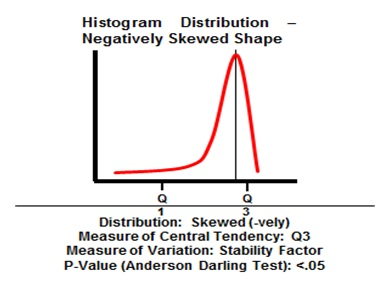Six Sigma Histogram Distribution Negatively Skewed ShapeSix Sigma Histogram Distribution Long Tailed ShapeSix Sigma Histogram Distribution Bi-Modal Symmetrical Shape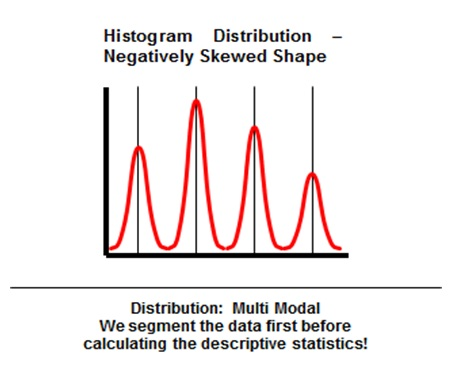Six Sigma Histogram Distribution Multi-Modal Negatively Skewed Shape

What do I do when data is Non Normal?
When the data is non-normal, we transform the data from non–normal to normal. We can transform the data with the following possibilities:
• Take the Log (any Base) of your raw measurement.
• Raise your individual measurements to a power.
• Use the reciprocal (1/y) of your individual measurements.
When we transform data, we should take following caution:
• When you transform your data, you must also transform your specification limits.
• You will use the transformed data throughout the analysis of your data.
• Use transformation of data the last resort after checking all other alternatives such as more data collection, validating data consistency.

# We guarantee that Your Free Online Training will make you pass Your Six Sigma Certification Exam!